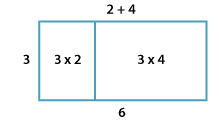### The distributive law – multiplication over addition

3 (2 + 4) = 3 × 2 + 3 × 4More generally,

a × (b + c) = (a × b) + (a × c) for any numbers a, b and c.

We use the distributive law to simplify some multiplication problems. We will return to consider more of these when we consider the distributive law for multiplication over subtraction.

For example:

• 7 × 101 = 7 × (100 + 1) = 700 + 7 = 707
• 7 × 102 = 7 × (100 + 2) = 700 + 14 = 714.